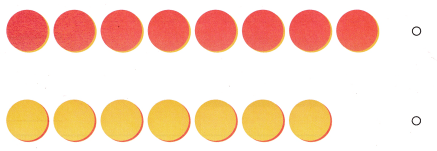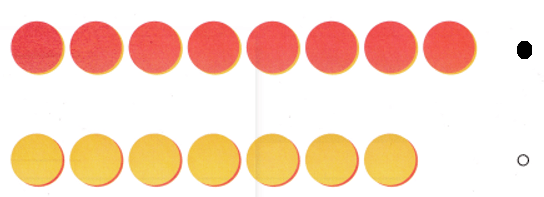# Texas Go Math Kindergarten Lesson 6.2 Answer Key Compare by Matching Sets to 10

Refer to our Texas Go Math Kindergarten Answer Key Pdf to score good marks in the exams. Test yourself by practicing the problems from Texas Go Math Kindergarten Lesson 6.2 Answer Key Compare by Matching Sets to 10.

## Texas Go Math Kindergarten Lesson 6.2 Answer Key Compare by Matching Sets to 10

Explore

DIRECTIONS: Make a cube train with 3 cubes. Make a cube train with 6 cubes. Compare the cube trains. Draw the cubes. Circle the set that has fewer cubes.Explanation:
cube train with 3 cubes < cube train with 6 cubes.

Share and Show
DIRECTIONS: 1. Make red and blue cube trains to model the numbers of balloons in the sets. Compare the cube trains by matching. Draw and color the cube trains. Write how many in each set. Circle the cube train that has more cubes.

Question 1.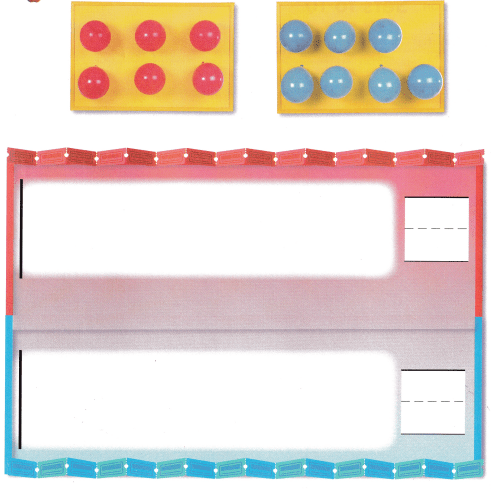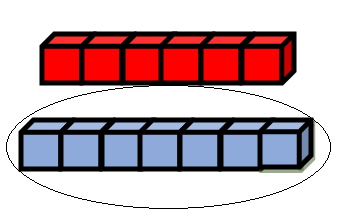Explanation:
Number of red cubes = 6.
Number of blue cubes = 7.
=> 6 < 7.

DIRECTIONS: 2. Make a 5-cube train and a 7-cube train. Compare the cube trains by matching. Draw and color the cube trains. Write how many. Circle the cube train with fewer cubes. 3. Make a 6-cube train and a 9 cube train. Compare the cube trains by matching. Draw and color the cube trains. Write how many. Circle the cube train with fewer cubes.

Question 2.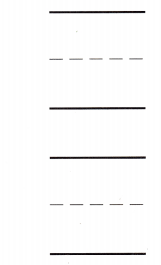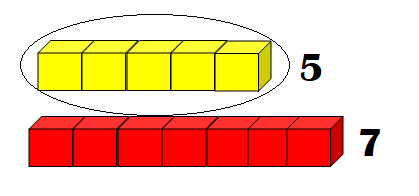Explanation:
Number of yellow cubes = 5.
Number of red cubes = 7.
5 < 7.

Question 3.Explanation:
Number of Green cubes = 6.
Number of yellow cubes = 9.
6 < 9.

HOME ACTIVITY • Ask your child to show two sets of up to 10 objects each. Then have him or her compare the sets by matching and tell which set has more objects.
Number of pens set has more objects than Number of pencils.

Explanation:
My child took pencils and pens.
Number of pencils = 6.
Number of pens = 10.
6 < 10.

DIRECTIONS: 4. Look at the cube train. Are there more blue cubes or more red cubes? Make cube trains of each color. Compare the cube trains by matching. Draw and color the cube train that has more cubes. Write the number. 5. Choose the correct answer. Which cube train has more cubes?

Problem Solving
Question 4.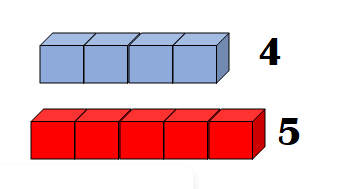Explanation:
Number of blue cubes = 4.
Number of red cubes = 5.
4 < 5.

Question 5.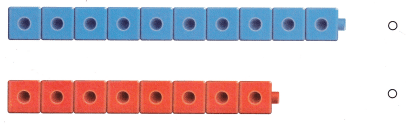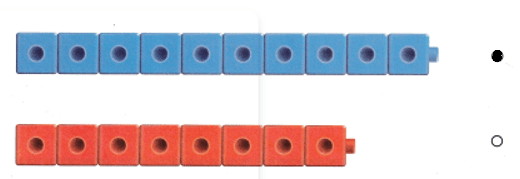Explanation:
Number of blue cubes = 10.
Number of red cubes = 8.
10 > 8.

### Texas Go Math Kindergarten Lesson 6.2 Homework and Practice Answer Key

DIRECTIONS: 1-2. Compare the cube trains by matching. Write how many. Circle the cube train with fewer cubes.

Question 1.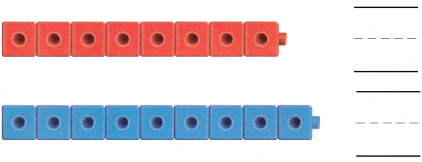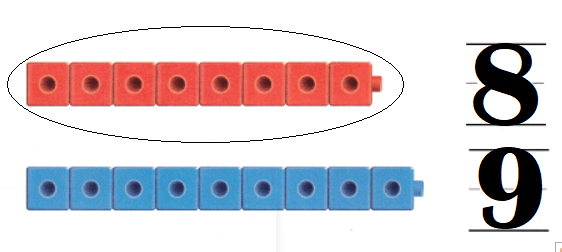Explanation:
Number of red cubes = 8.
Number of blue cubes = 9.
8 < 9.
Question 2.Explanation:
Number of yellow cubes = 10.
Number of green cubes = 6.
10 > 6.

DIRECTIONS: Choose the correct answer. 3-4. Are there more red counters or yellow counters?

Lesson Check
Question 3.Explanation:
Number of red dots = 4.
Number of yellow dots = 6.
4 < 6.

Question 4.